StatLect

Countable additivity is one of the properties that characterize probability.## Synonyms

Countable additivity is also called sigma-additivity (-additivity).

## The property

A well-defined probability measuremust have the property that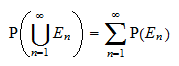where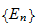is a sequence of mutually exclusive events (i.e.,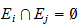if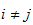).

In other words, the probability of a countable union of disjoint events must be equal to the sum of their probabilities.

This property is called countable additivity.

It is easy to prove that countable additivity implies finite additivity, that is,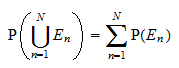for any set ofmutually exclusive events.

Proof

Note that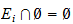for any. Therefore, we can set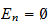forin the definition of countable additivity.

## How can it be proved?

It cannot be proved. It is a defining property of probability. It is an axiom thatis required to satisfy in order to be called a probability.

## Why is it required?

Why don't we impose the simpler and more easily understandable axiom of finite additivity?

Basically, it is for mathematical convenience.

With a slight abuse of notation, we would like the following continuity property of probability to hold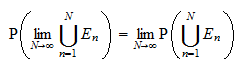which allows us to interchange limits and probabilities.

This continuity is guaranteed only by the sigma-additivity axiom.

## More details

The lecture on probability contains more details about countable additivity and the other two properties that define probability, namely:

• the range property,

• the sure thing property.

Previous entry: Convolutions

Next entry: Covariance formula